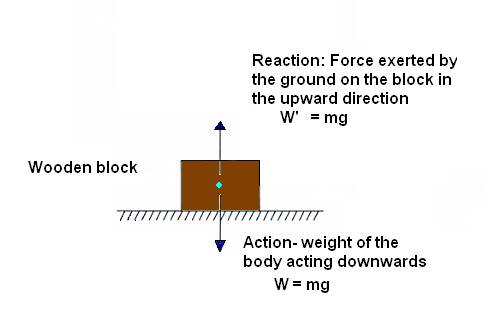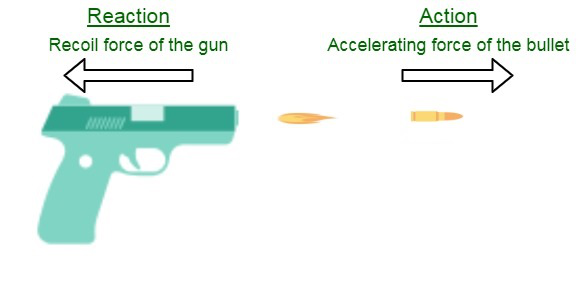Crystal

MS-PS2-1

# Newton’s Third Law Study Guide

The action and reaction law by Newton is one of the most powerful laws on the planet and defines almost all of our daily activities.

# Introduction

Have you ever thought how simple acts like walking can be a spectacular demonstration of one of physics' most important laws by Newton? When we dissect the fundamental process of walking, we can see that when we take a step, we press our foot against the ground, which causes us to move ahead. Let's dive deep into this amazing physics law to better understand the concept.Source

## MOTION

• Before we can comprehend what Newton has to say about his laws, we must first understand what motion is.
• In physics, the meaning of motion is the change in the location of an object in relation to its surroundings during a period of time.
• The motion of a massed item can be explained by acceleration, displacement, distance, speed, time, and velocity.
• Newton's Laws of Motion ( first, second and third law) set the groundwork for what is now known as classical mechanics.
• These physical laws are applicable every day and are consequently employed, despite slight limits.
• Here we’ll talk about the third law of motion, popularly known as the law of action and reaction.

## THE THIRD LAW OF MOTION

• Newton’s third law states, ‘to every action, there is an equal and opposite reaction.’
• In other words, if item H exerts a force on object O, object O must respond with a force of similar magnitude and in the reverse direction.
• This can be mathematically represented by the formula of Newton’s third law of motion as

Fh ( force exerted by an object h on object o ) = - Fo( force exerted by the object o on object h)

It is also important to note that as the action and reaction forces act on different bodies, they can not cancel out each other.Source

This law can be noticed in the surroundings at any time and in any place. The following are some examples of the third Law.

• While flying, a bird uses its wings to force the air downward, thus making it fly upwards.

• A swimmer uses his hands to move the water backward, and the water, in return, drives the person ahead.

# SUMMARY

• Motion is defined as a change in the location of an object.
• Newton’s third law states that “to every action, there is an equal and opposite reaction.”
• Action and reaction forces act on different bodies, and they can not cancel out each other.

## FAQs

1. What is the mathematical representation of Newton’s third law of motion?

The mathematical representation of Newton’s third law of motion is Fh = - Fo

2. Mention some examples of interaction force pairs.

Everyday activities like walking, skateboarding, or even writing are examples of Interaction Force Pairs.

3. State Newton’s 3rd law of motion?

Newton’s third law states, ‘to every action, there is an equal and opposite reaction.’

We hope you enjoyed studying this lesson and learned something cool about Newton’s Third Law! Join our Discord community to get any questions you may have answered and to engage with other students just like you! We promise, it makes studying much more fun!😎

## REFERENCE

1. Newton’s Third Law: https://flexbooks.ck12.org/cbook/ck-12-middle-school-physical-science-flexbook-2.0/section/11.6/primary/lesson/newtons-third-law-ms-ps/. Accessed 8th April 2022.
2. Newton’s Law of Motion: https://en.wikipedia.org/wiki/Newton%27s_laws_of_motion. Accessed 8th April 2022